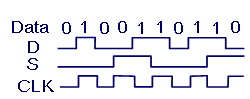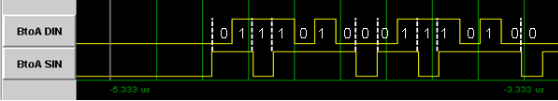SpaceWire uses Data-Strobe (DS) encoding. This is a coding scheme which encodes the transmission clock with the data into Data and Strobe so that the clock can be recovered by simply XORing the Data and Strobe lines together. The data values are transmitted directly and the strobe signal changes state whenever the data remains constant from one data bit interval to the next. This coding scheme is illustrated in Figure 30. The DS encoding scheme is also used in the IEEE 1355-1995 and IEEE 1394-1995 (Firewire) standard.

The reason for using DS encoding is to improve the skew tolerance to almost 1-bit time, compared to 0.5 bit time for simple data and clock encoding.Figure 30 Data-Strobe (DS) Encoding

A SpaceWire link comprises two pairs of differential signals, one pair transmitting the D and S signals in one direction and the other pair transmitting D and S in the opposite direction. That is a total of eight wires for each bi-directional link.

A bit trace from a SpaceWire link analyser is shown in Figure 31. The yellow lines are the data and strobe signals (DIN and SIN). At each transition of the strobe line a dotted white line has been drawn vertically from the strobe signal over the data signal. The value of the data line between these vertical lines or the transitions of the data line is the decoded data value, shown as white 0 or 1 on the trace. Bit synchronisation on SpaceWire is therefore quite straightforward, but determining the boundaries between SpaceWire characters is more difficult in a bit trace.Figure 31 Decoding SpaceWire Data

SpaceWire performs bit synchronisation by XORing the data and strobe signals to form a clock signal, both edges of which are then used to read in the values on the data line.